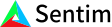1. Bob Anderson
×
IntuitiveML

## Loss Functions: What are they and why are they important?

Jul 4, 2020

Loss functions tell us how wrong our predictions are during training. We then use that information to optimize our machine learning model. But - wait, what about accuracy, precision, and recall - can’t we use those to figure out how wrong our predictions are?

While we can use metrics such as accuracy to get an idea of how wrong our predictions are and to compare various methods and models, they often fail for use during optimization because they aren’t differentiable. That is, a lot of machine learning methods use gradient based optimizers, which just means that the function they optimize has to be differentiable in order to learn. Accuracy, precision, and recall aren’t differentiable, so we can’t use them to optimize our machine learning models.

A loss function is any function used to evaluate how well our algorithm models our data. The higher the loss, the worse our model is performing. We then try to minimize that function in order to to ‘learn’ how to solve the task at hand. In supervised learning, most loss functions compare the predicted output with the label. That is, most loss functions measure how far off our output was from the actual answer.

For example, if you are trying to classify whether or not a picture has a dog in it (0 not a dog, 1 dog), your algorithm might output .6. After rounding, you see that you predicted this was a dog. However, during training we are trying to get better and better predictions, e.g. if something is a dog, then we want the algorithm to output as close to 1 as possible. A loss function that might make sense is to take the absolute value of the difference – i.e. the loss is equal to exactly how far off our prediction is. In the dog example, this loss would be .4. You then modify your model based on the size of your loss – if you have a high loss, it will change more than when you have a low loss. As a side note, it’s important to choose a good loss function – if you penalize the wrong things, then your model could not learn at all or worse, learn the wrong thing.

In general, loss functions have two properties: they are globally continuous and differentiable. This basically just means that the function you use can’t jump, it is defined at every point, it has no sharp turns and no vertical tangents.

A useful property of most loss functions is that they are symmetric, that is for a loss function, the loss(actual_output, predicted_output) = loss(predicted_output, actual_output). Most of the time this is nice to have since it makes sense that the loss of something being actually zero but predicting it is one and the loss of something being actually one but predicting it is zero should be the same.

Overall, loss functions are just functions we use to measure our performance and optimize our machine learning models.